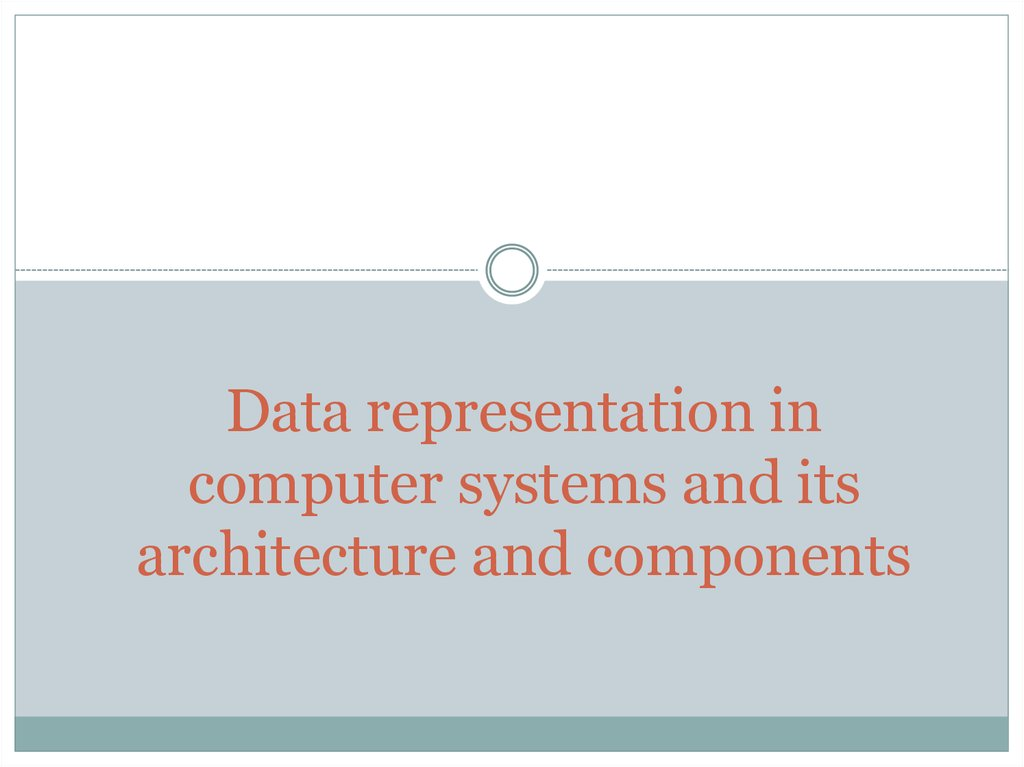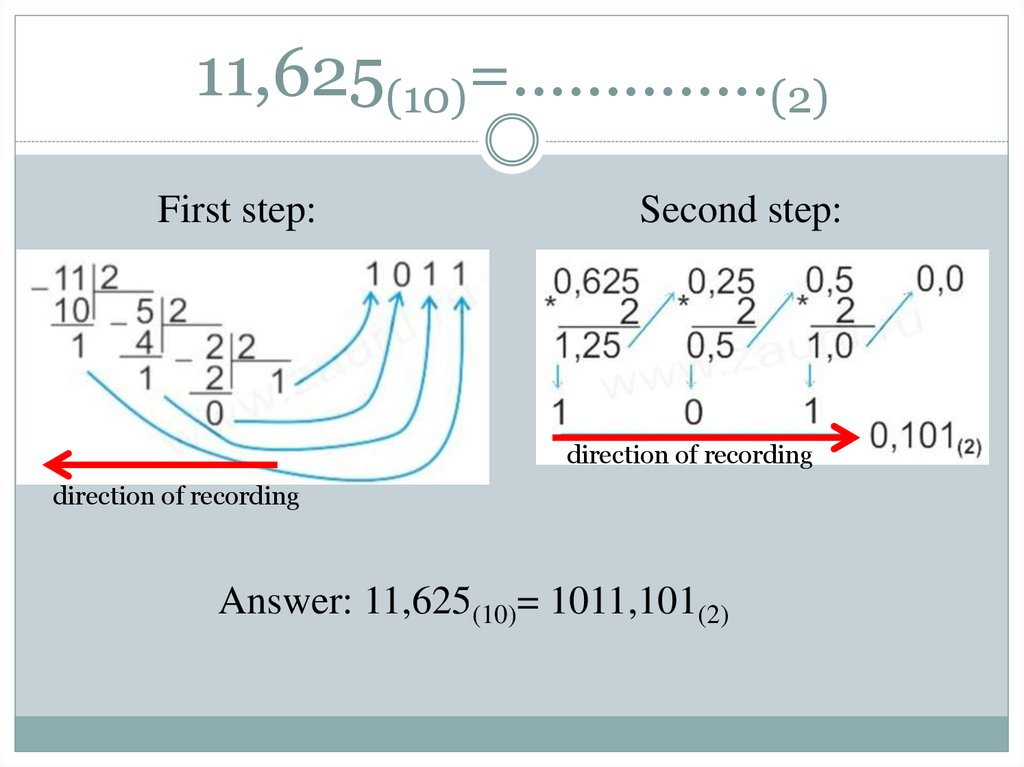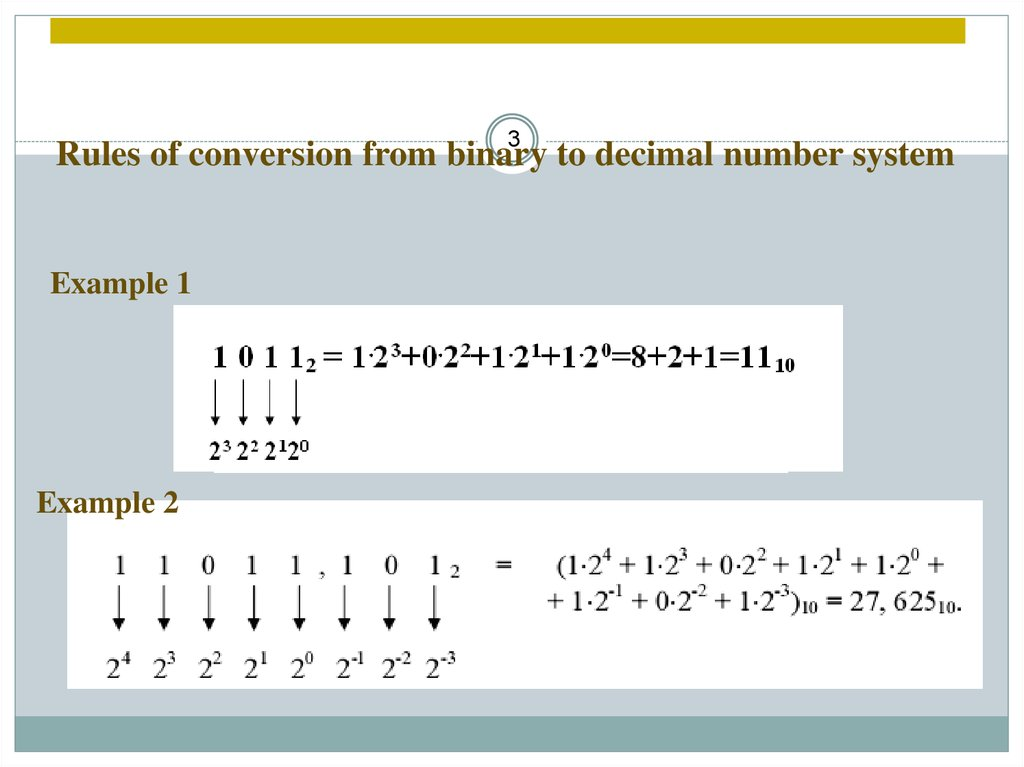# Data representation in computer systems and its architecture and components

## 1.

Data representation in
computer systems and its
architecture and components

## 2. 11,625(10)=…………..(2)

First step:
Second step:
direction of recording
direction of recording

## 3.

3
Rules of conversion from binary to decimal number system
Example 1
Example 2

## 4.

4 decimal to binary number
Rules of conversion from
system
conversion 345 to binary number system:
1

Find 110011+1101
5
0+0=0
1+0=1
0+1=1
1+1=1 0
1+1+1=1 1
1 1 1 1 1
110011
+
1101
1000000

## 6. 122,6(10)=…………..(8)

First step:
direction of recording
Second step:
direction of recording

## 7.

500,7(10)=…………..(16)
First step:
Second step:
direction of recording
direction of recording

## 8.

1. Converting binary to decimal